susceptance

(redirected from Permittance)
Also found in: Dictionary, Legal, Wikipedia.
Related to Permittance: Disingenuity, ebullience

Susceptance

The imaginary part of the admittance of an alternating-current circuit.

The admittance, Y, of an alternating current circuit is a complex number given by Eq. (1). The imaginary part, B, is the susceptance.

(1)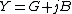The units of susceptance like those of admittance are called siemens or mhos. Susceptance may be either positive or negative. For example, the admittance of a capacitor C at frequency &ohgr; is given by Eq. (2), and so B is positive. For an inductor L, the admittance is given by Eq. (3), and so B is negative. (2)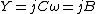(3)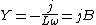In general, the susceptance of a circuit may depend on the resistors as well as the capacitors and inductors. For example, the circuit in the illustration has impedance given by Eq. (4) and admittance given by Eq. (5), so that the susceptance, given by Eq. (6), depends on the resistor R as well as the inductor L. (4)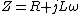(5)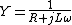(6)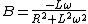See Admittance, Electrical impedance

susceptance

[sə′sep·təns]
(electricity)
The imaginary component of admittance.
Site: Follow: Share:
Open / Close